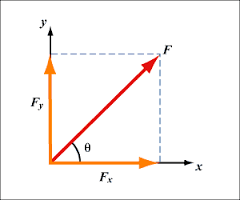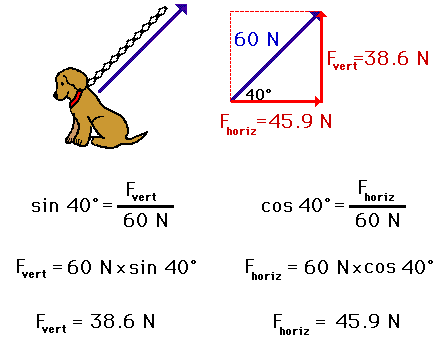## 2015-03-22

### Resolution of Forces

We know that two forces when combined together will form a single resultant force. On the other hand, a single force can be divided or broken up into two components.

The reversal of this process is called the resolution of forces. A force is usually resolved into two components that are perpendicular to each other.

A force can be resolved into two component forces graphically or by using trigonometry.Consider the diagram above. In the diagram the force F is resolved into two perpendicular component forces that is the Fy and Fx components (using parallelogram method).

To calculate the magnitude of the vertical (Fy) and horizontal (Fx) forces, we can use simple trigonometry.

Fx =  F cos θ , Since cos θ = (Fx/F)

Fy = F sin θ, Since sin θ = (Fy/ F)

Examples of calculation:

By using the diagram above, Let say F = 80 N and θ = 30 degree

The horizontal component Fx,
= F cos θ
= 80 cos 30
= 80 X 0.866
= 69.3 N to the right

The vertical component Fy,
= F sin θ
= 80 sin 30
= 80 x 0.5
= 40 N upwards

Another example would be as below:Source: imgkid.com

Another good example:Source: Physicsclassroom.com

#### 2 comments:

Mobile App Developers said...

This is the precise weblog for anybody who needs to seek out out about this topic. You notice so much its almost arduous to argue with you. You positively put a brand new spin on a subject that's been written about for years. Nice stuff, simply nice!

John said...

Very useful for students